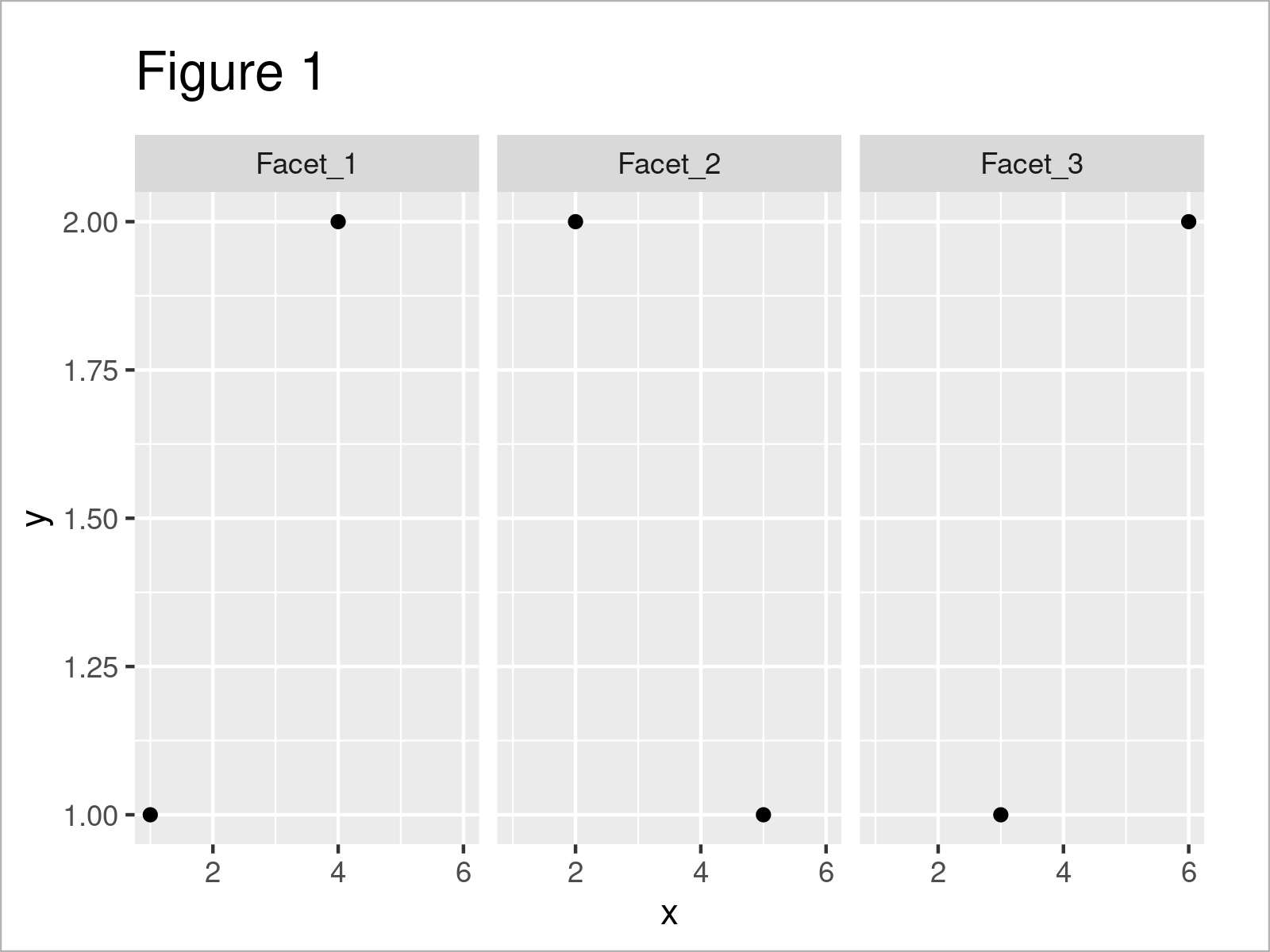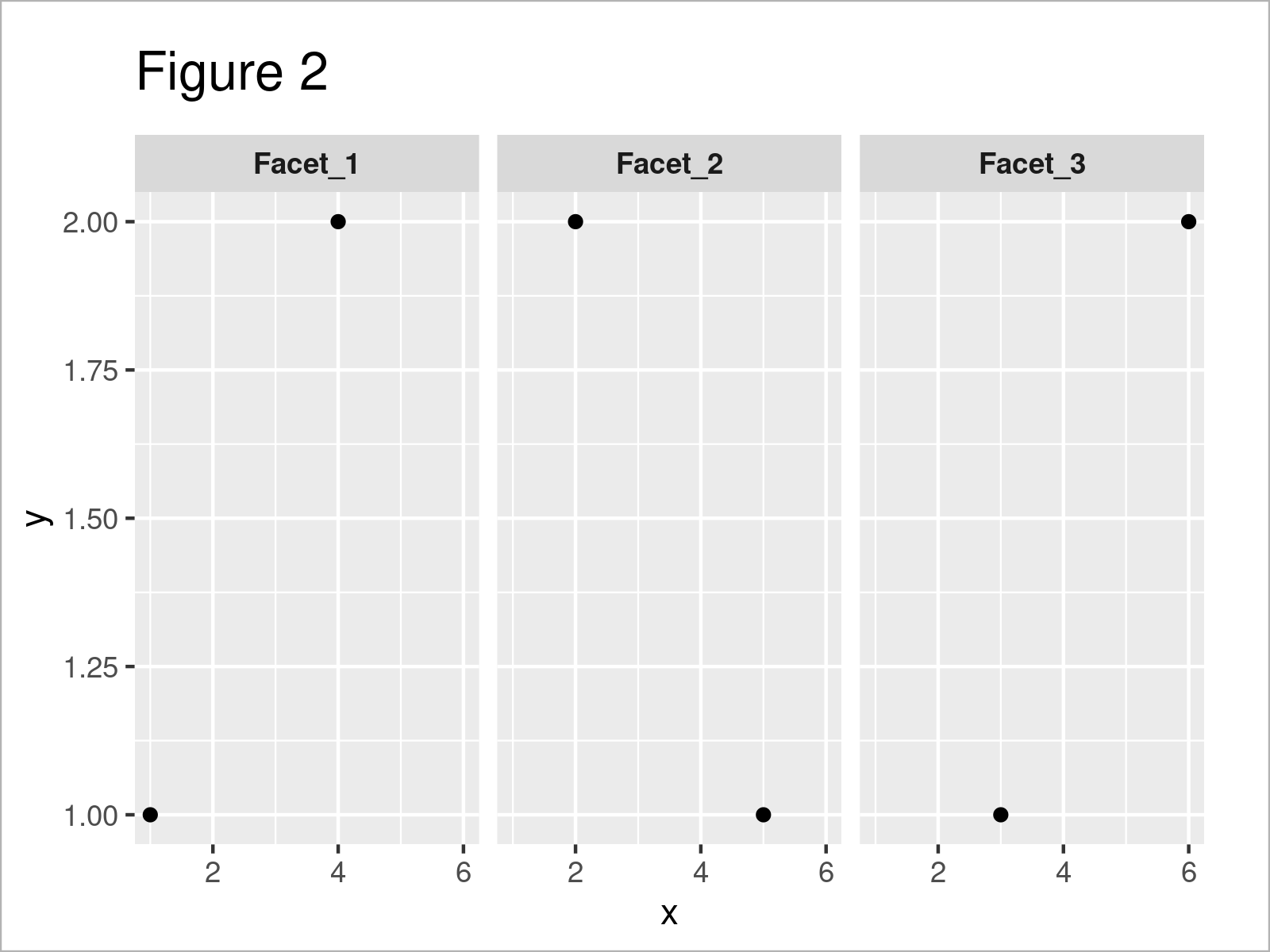# Display Labels of ggplot2 Facet Plot in Bold or Italics in R (2 Examples)

On this page, I’ll show how to change the labels of a ggplot2 facet plot to bold or italics in the R programming language.

The tutorial contains the following contents:

Let’s get started!

## Example Data, Packages & Basic Plot

The first step is to create some data that we can use in the following examples.

```data <- data.frame(x = 1:6,         # Create example data frame
y = 1:2,
group = paste0("Facet_", 1:3))
data                                # Print example data frame```Table 1 reveals the structure of our example data: It is composed of six rows and three columns. The variables x and y are integers and the variable group has the character class.

We also have to install and load the ggplot2 package, in order to use the corresponding functions:

```install.packages("ggplot2")         # Install & load ggplot2 package
library("ggplot2")```

Now, we can create a graphic of our data as shown below:

```ggp <- ggplot(data, aes(x, y)) +    # Create ggplot2 facet plot
geom_point() +
facet_grid(. ~ group)
ggp                                 # Draw ggplot2 facet plot```Figure 1 reveals the output of the previous R syntax: A ggplot2 facet graphic with default facet labels.

Note that we have used the facet_wrap function to create our facet plot. However, we could also use the facet_grid function for this.

## Example 1: Display Labels of ggplot2 Facet Plot in Bold

The following R syntax explains how to change the labels of a ggplot2 facet graph to bold.

For this task, we can use the theme function as shown below:

```ggp +                               # Change labels to bold
theme(strip.text = element_text(face = "bold"))```The output of the previous R programming syntax is shown in Figure 2 – Our facet labels have been converted to bold.

## Example 2: Display Labels of ggplot2 Facet Plot in Italics

This example shows how to display ggplot2 facet plot labels in italics.

Once again, we can use the theme function for this task:

```ggp +                               # Change labels to italics
theme(strip.text = element_text(face = "italic"))```After running the previous R syntax the ggplot2 facet plot with italic labels shown in Figure 3 has been created.

## Video, Further Resources & Summary

In case you need further explanations on the R programming syntax of this article, you may want to have a look at the following video that I have published on my YouTube channel. In the video, I explain the topics of this article:

Please accept YouTube cookies to play this video. By accepting you will be accessing content from YouTube, a service provided by an external third party.If you accept this notice, your choice will be saved and the page will refresh.

This tutorial has explained how to change all text labels of a ggplot2 facet plot to bold or italics. However, it’s also possible to change only one specific label. Have a look here for more details.

Furthermore, you may read some of the related tutorials that I have published on my homepage:

In summary: In this R programming article you have learned how to convert the labels of a ggplot2 facet graphic to bold or italics. Please let me know in the comments section below, in case you have any additional questions.

Subscribe to the Statistics Globe Newsletter